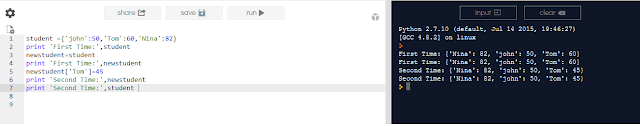## Blog Pages

### New Dictionary Creation and updation

student ={'john':50,'Tom':60,'Nina':82}
print 'First Time:',student
newstudent=student
print 'First Time:',newstudent
newstudent['Tom']=45
print 'Second Time:',newstudent
print 'Second Time:',student

online compiler used  https://repl.it/languages/python### Transpose of a matrix (nested list) in python

row1 = [13,25,73]
row2 = [54,95,36]
row3 = [27,98,19]

matrix = [row1, row2, row3]
trmatrix = [[row for row in matrix],[row for row in matrix],  [row for row in matrix]]
print'Transpose of a matrix',trmatrix

source: http://stackoverflow.com